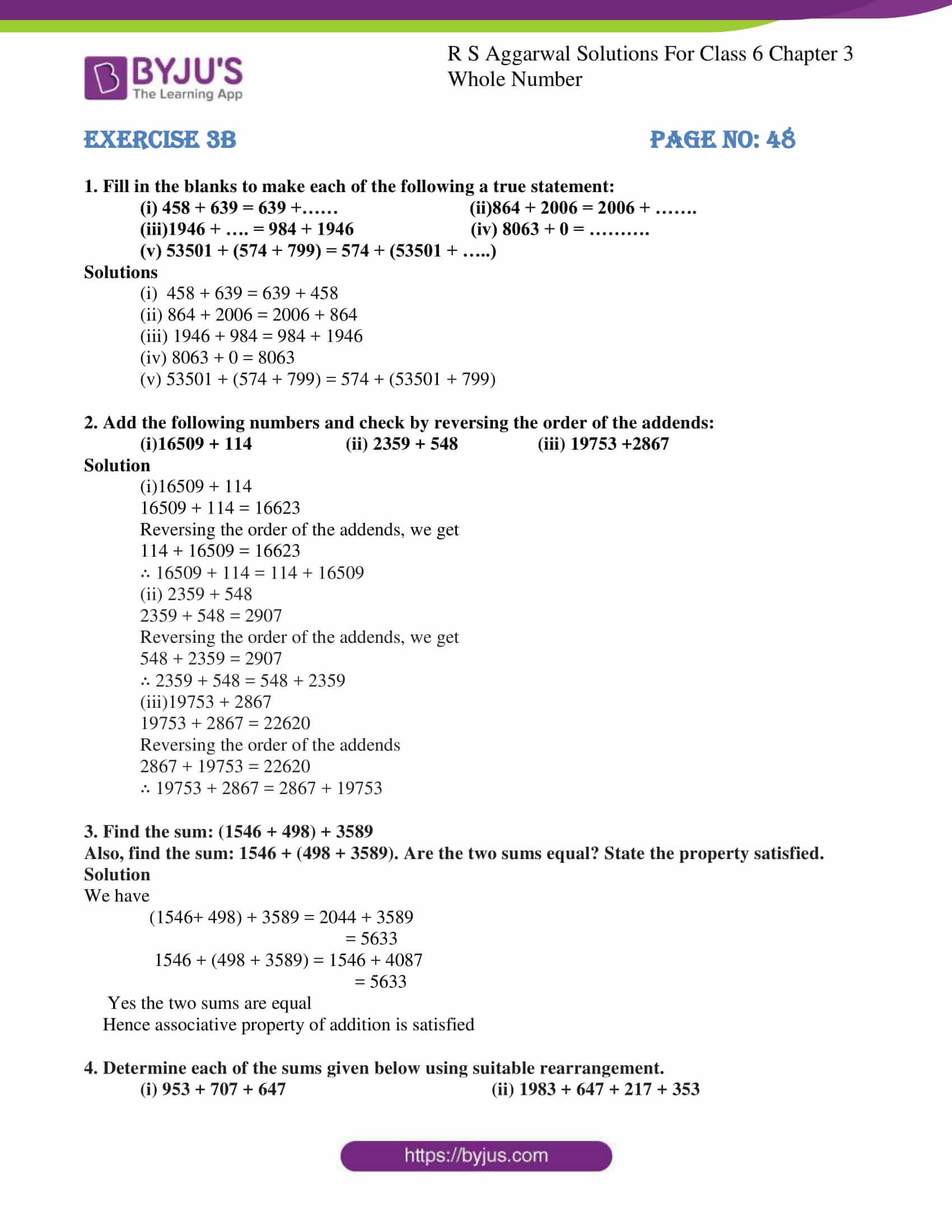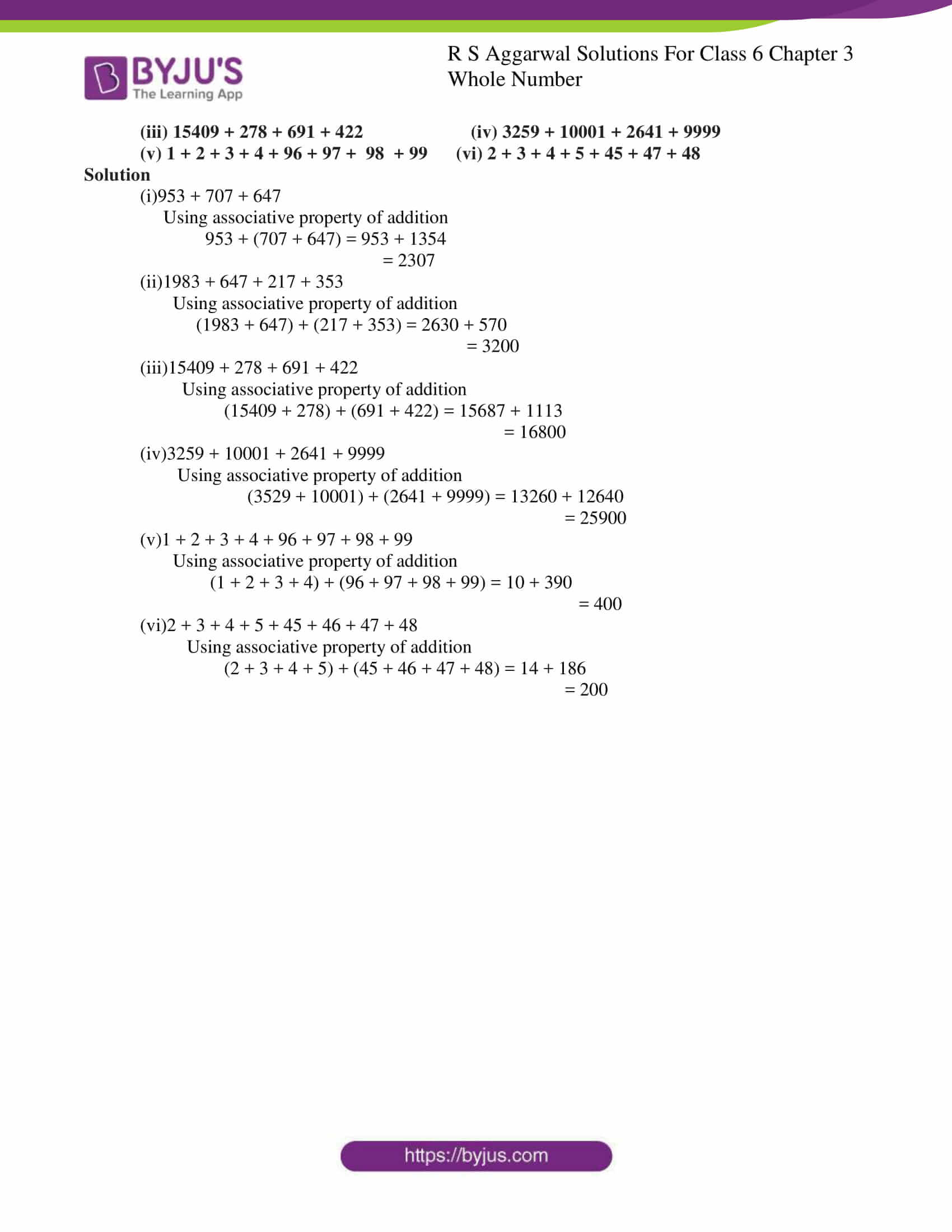# RS Aggarwal Solutions for Class 6 Chapter 3 Whole Numbers Exercise 3B

RS Aggarwal Solutions for Class 6, Chapter 3, Whole Numbers Exercise 3B are available in PDF here. This Exercise 3B of RS Aggarwal Solutions for Class 6 Chapter 3 Whole Numbers has questions related to operations on Whole Numbers and properties of addition like Closure property, Commutative law, Additive property of zero and Associative law. Students are advised to go through RS Aggarwal Solutions for Class 6 Chapter 3 Whole Numbers Exercise 3B and practice to learn these concepts more effectively.

## Download PDF of RS Aggarwal Solutions for Class 6 Chapter 3 Whole Numbers Exercise 3B### Access answers to Maths RS Aggarwal Solutions for Class 6 Chapter 3 Whole Numbers Exercise 3B

1. Fill in the blanks to make each of the following a true statement:

(i) 458 + 639 = 639 +…… (ii)864 + 2006 = 2006 + …….

(iii)1946 + …. = 984 + 1946 (iv) 8063 + 0 = ……….

(v) 53501 + (574 + 799) = 574 + (53501 + …..)

Solutions

(i) 458 + 639 = 639 + 458

(ii) 864 + 2006 = 2006 + 864

(iii) 1946 + 984 = 984 + 1946

(iv) 8063 + 0 = 8063

(v) 53501 + (574 + 799) = 574 + (53501 + 799)

2. Add the following numbers and check by reversing the order of the addends:

(i)16509 + 114 (ii) 2359 + 548 (iii) 19753 +2867

Solution

(i)16509 + 114

16509 + 114 = 16623

Reversing the order of the addends, we get

114 + 16509 = 16623

∴ 16509 + 114 = 114 + 16509

(ii) 2359 + 548

2359 + 548 = 2907

Reversing the order of the addends, we get

548 + 2359 = 2907

∴ 2359 + 548 = 548 + 2359

(iii)19753 + 2867

19753 + 2867 = 22620

Reversing the order of the addends

2867 + 19753 = 22620

∴ 19753 + 2867 = 2867 + 19753

3. Find the sum: (1546 + 498) + 3589

Also, find the sum: 1546 + (498 + 3589). Are the two sums equal? State the property satisfied.

Solution

We have

(1546+ 498) + 3589 = 2044 + 3589

= 5633

1546 + (498 + 3589) = 1546 + 4087

= 5633

Yes the two sums are equal

Hence associative property of addition is satisfied

4. Determine each of the sums given below using suitable rearrangement.

(i) 953 + 707 + 647 (ii) 1983 + 647 + 217 + 353

(iii) 15409 + 278 + 691 + 422 (iv) 3259 + 10001 + 2641 + 9999

(v) 1 + 2 + 3 + 4 + 96 + 97 + 98 + 99 (vi) 2 + 3 + 4 + 5 + 45 + 47 + 48

Solution

(i)953 + 707 + 647

953 + (707 + 647) = 953 + 1354

= 2307

(ii)1983 + 647 + 217 + 353

(1983 + 647) + (217 + 353) = 2630 + 570

= 3200

(iii)15409 + 278 + 691 + 422

(15409 + 278) + (691 + 422) = 15687 + 1113

= 16800

(iv)3259 + 10001 + 2641 + 9999

(3529 + 10001) + (2641 + 9999) = 13260 + 12640

= 25900

(v)1 + 2 + 3 + 4 + 96 + 97 + 98 + 99